# Elimination method

Solve system of linear equations by elimination method:

5/2x + 3/5y= 4/15
1/2x + 2/5y= 2/15

x =  0.0381
y =  0.2857

### Step-by-step explanation:

5/2•x + 3/5•y= 4/15
1/2•x + 2/5•y= 2/15

75x+18y = 8
15x+12y = 4

x = 4/105 ≈ 0.038095
y = 2/7 ≈ 0.285714

Our linear equations calculator calculates it.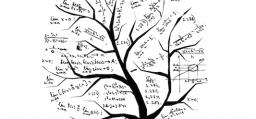Did you find an error or inaccuracy? Feel free to write us. Thank you!Tips to related online calculators
Do you have a system of equations and looking for calculator system of linear equations?

## Related math problems and questions:

• EquationsSolve following system of equations: 6(x+7)+4(y-5)=12 2(x+y)-3(-2x+4y)=-44
• Inverse matrix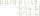Find out inverse by Gauss elimination or by reduction method. A=[2/3. 1 -3. 1/3]
• Two equationsSolve equations (use adding and subtracting of linear equations): -4x+11y=5 6x-11y=-5
• Linear systemSolve a set of two equations of two unknowns: 1.5x+1.2y=0.6 0.8x-0.2y=2
• Median or middle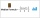The number of hours of television watched per day by a sample of 28 people is given below: 4, 1, 5, 5, 2, 5, 4, 4, 2, 3, 6, 8, 3, 5, 2, 0, 3, 5, 9, 4, 5, 2, 1, 3, 4, 7, 2, 9 What is the median value?
• Land cost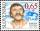The land is worth 33295 CZK. 1st owner has 5/15, 2nd owner has 4/15, 3rd owner has 1/5 4th owner has 1/5. How much CZK each of them will receive?
• ComplaintsThe table is given: days complaints 0-4 2 5-9 4 10-14 8 15-19 6 20-24 4 25-29 3 30-34 3 1.1 What percentage of complaints were resolved within 2weeks? 1.2 calculate the mean number of days to resolve these complaints. 1.3 calculate the modal number of day
• Three unknownsSolve the system of linear equations with three unknowns: A + B + C = 14 B - A - C = 4 2A - B + C = 0Which of the points belong function f:y= 2x2- 3x + 1 : A(-2, 15) B (3,10) C (1,4)
• Rajendra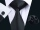Rajendra a farmer had two sons and two daughters. He decided to divide his property among his sons and daughters. So he wrote a "WILL" about distribution of his property. According to his "WILL", he desired to give 3/5 th of the property to his sons in eq
• Percent to interval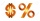76% is between which of the following two numbers. 1) 1/5 & 1/4. 2) 1/3 & 1/2 3) 2/3 & 7/10 4) 3/4 & 5/6
• Sequence 11What is the nth term of this sequence 1, 1/2, 1/3, 1/4, 1/5?
• Three points 4The line passed through three points - see table: x y -6 4 -4 3 -2 2 Write line equation in y=mx+b form
• Watching TVOne evening 2/3 of students watch TV. Of those students, 3/8 watched a reality show. Of the students that watched the show, 1/4 of them recorded it. What fraction of the students watched and recorded reality tv.
• Equations - simpleSolve system of linear equations: x-2y=6 3x+2y=4
• Non linear eqsSolve the system of non-linear equations: 3x2-3x-y=-2 -6x2-x-y=-7
• Evaluate mixed expressionsWhich of the following is equal to 4 and 2 over 3 divided by 3 and 1 over 2? A. 4 and 2 over 3 times 3 and 2 over 1 B. 14 over 3 times 2 over 7 C. 14 over 3 times 7 over 2 D. 42 over 3 times 2 over 31